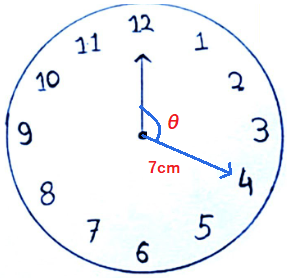mycollegehive
The length of the minute hand of a clock is 7cm. How much area does it sweep in 20minutes
CBSE class-10 math areas-related-to-circles0The length of the minute hand of a clock is 7cm. How much area does it sweep in 20minutes

16 viewsShareFollowSchool not set Nigeria
31 July 2020University of Lagos Nigeria
31 July 20200The angle round a complete circle is 360.

It takes 60 minutes for the minute hand to competely move round the clock.

If in 20 minutes, the minute hand makes angle θ with the hour hand.

To find θ, we compare the minutes and angle covered by the minute hand to the complete standard minutes and angle. That is,

20/60 = θ/360

1/3 = θ/360

3θ = 360

divide both sides by 3

θ = 120

Thus, in 20 minutes, the minute hand will make angle 120 with the hour hand.

Area of the sector = 1/2 (length of arc x r)

length of arc = θ/360 x 2πr

Thus, area of the sector = 1/2 (θ/360 x 2πr x r)

= 1/2 (θ/360 x 2πr2)

= (θ/360 x πr2)

The length of the minute hand represents the radius of the circle.

= 120/360 x π(7)2

= 49π/3

but  π = 22/7

= 49/3 x 22/7

= 154/3Share### Related Tags

CBSE

0 followers

56 questionsclass-10

0 followers

56 questionsmath

2 followers

985 questions